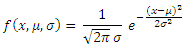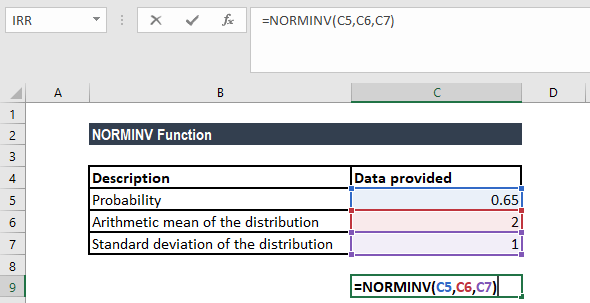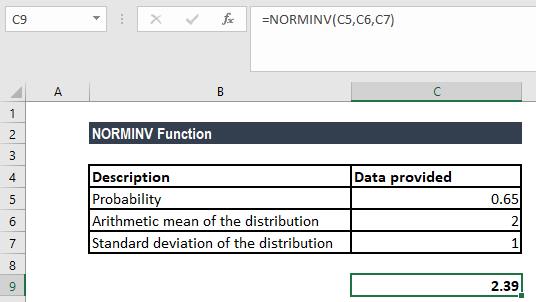# NORMINV Function

Calculates the inverse of the normal cumulative distribution for the specified mean and standard deviation

## What is the NORMINV Function?

The NORMINV Function is categorized under Excel Statistical functions. It will calculate the inverse of the normal cumulative distribution for the specified mean and standard deviation.

Suppose we are given a normally distributed random variable that is denoted by x. For the value of x, if we wish to get the bottom 10% of the distribution, we can use the NORMINV function.

As a financial analyst, NORMINV is useful in stock market analysis. We can use the function to understand how a portfolio is affected by any additions or withdrawals made.

### Formula

=NORMINV(probability,mean,standard_dev)

The NORMINV formula uses the following arguments:

1. Probability (required argument) – This is the probability corresponding to the normal distribution. It is the value at which we want to evaluate the inverse function.
2. Mean (required argument) – This is the arithmetic mean of the distribution.
3. Standard_dev (required argument) – The standard deviation of the distribution.

The Normal distribution is a continuous probability function and is given by the formula:Where:

• μ is the mean of the distribution
• σis the variance
• is the independent variable for which we want to evaluate the function.

### How to use the NORMINV Function in Excel?

To understand the uses of the NORMINV function, let’s consider an example:

#### Example

Suppose we are given the following data:

• Probability: 65
• Arithmetic mean of the distribution: 2
• Standard deviation of the distribution: 1

If we wish to calculate the value of the function, the formula will be:We get the result below:### Things to remember about the NORMINV Function

1. In MS Excel 2010, the NORMINV function was replaced by the NORM.INV function. The function is still available in Excel 2010 and is stored in the list of compatibility functions to allow compatibility with earlier versions of the application.
2. NORMINV uses an iterative search technique. If the search fails to converge after 100 iterations, the function will return the #N/A error value.
3. #VALUE! error – Occurs if any of the given arguments is non-numeric.
4. #NUM! error – Occurs when:
• The given probability argument is less than zero or greater than 1.
• The given standard_dev argument is less than or equal to zero.

Thanks for reading CFI’s guide to important Excel functions! By taking the time to learn and master these functions, you’ll significantly speed up your financial analysis. To learn more, check out these additional CFI resources:

• Excel Functions for Finance